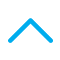To Buy: 1800-258-5899 (9 am to 6 pm)

|customerservice@canarahsbclife.in

|Locate Branch

# FAQs

### Frequently Asked Questions

##### What Is The Procedure To Calculate The Capital Gain Tax In India?

Capital gains are described as the profits that you accrue or receive through the sale of capital assets. When you sell any capital asset for an amount, more than you paid for it, your sale accrues capital gains. There are two types of capital gains – long-term and short-term.

Long-term capital assets are those, which you hold for 36 months or more. On the other hand, short-term assets are held for shorter durations. These capital assets include investment products such as stocks or mutual funds, and real estate products including land or house.

Tax on Capital Gains

The calculation of tax depends upon the type of capital gain accrued. Thus, the tax calculation for short-term capital gains is much simpler that on long-term capital gains.

1. Tax on Short-Term Gains

In case of short-term capital gains, you can add the gain amount to your total annual income and then calculate the income tax based on the tax bracket you fall in.

2. Tax on Long-Term Gains

When it comes to long-term capital gains, you have to consider other variables such as inflation (or cost inflation index) and indexation, while computing tax on long-term gains.

What is Cost Inflation Index (CII)?

Cost inflation Index or CII is a fixed value index that depicts the overall rate of inflation throughout the year, and is declared every year by the government of India.

What is Indexation?

Indexation is described as the process of adjusting prices of different assets based on a standard index. Doing this, helps factor-in the inflation rate while calculating the profits earned on sale of capital assets. Indexation is crucial since the prices tend to vary with time and do not remain flat. Hence, if you compute the profits based on the original price of a capital asset, it may not be an accurate measure.

Formulae for Calculating Capital Gains in India

1. To calculate Short-term Capital Gains (STCG) Tax

The computation for STCG is given below:

Short Term Capital Gain= Full Value Consideration – (Cost of Acquisition + Cost of Improvement + Cost of Transfer)

2. To Calculate Long-Term Capital Gain (LTCG) Tax

Long Term Capital Gain = Full Value of Consideration Received or Accruing – (Indexed Cost of Acquisition + Indexed Cost of Improvement + Cost of Transfer)

Here;

Indexed cost of acquisition = (Cost of Acquisition x Cost Inflation Index of the year of transfer) / (Cost Inflation Index of the year of acquisition)

Indexed Cost of Improvement = (Cost of Improvement x Cost Inflation Index of the year of transfer) / (Cost Inflation Index of the year of improvement)